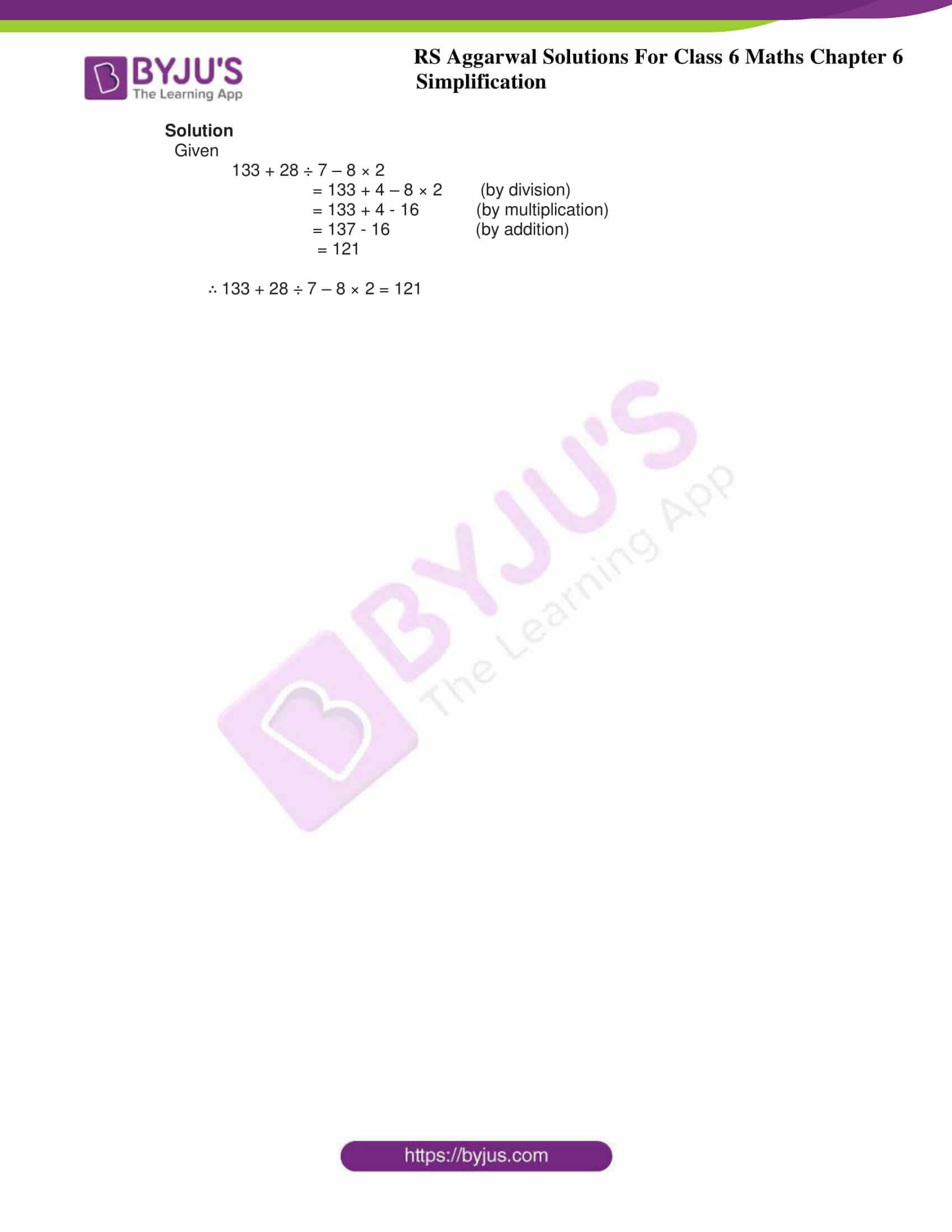# RS Aggarwal Solutions for Class 6 Chapter 6 Simplification Exercise 6B

RS Aggarwal Solutions for Class 6 Chapter 6 Simplification Exercise 6B consists of objective type questions with answers designed as per CBSE guidelines. These types of questions help the students to have a better hold of the concepts effectively. By solving these questions on a daily basis, the students can find the concepts which require more practice. RS Aggarwal Solutions solved by experts help students expertise in Mathematics.

## Download PDF of RS Aggarwal Solutions for Class 6 Chapter 6 Simplification Exercise 6B### Access answers to Maths RS Aggarwal Solutions for Class 6 Chapter 6 Simplification Exercise 6B

OBJECTIVE QUESTIONS

1. 8 + 4 ÷ 2 × 5 = ?

(a) 30 (b) 50 (c) 18 (d) none of these

Solution

Given

8 + 4 ÷ 2 × 5

= 8 + 2 × 5 (by dividing)

= 8 + 10 (by multiplying)

= 18

∴ 8 + 4 ÷ 2 × 5 = 18

2. 54 ÷ 3 of 6 + 9 = ?

(a) 117 (b) 12 (c) 6 / 5 (d) none of these

Solution

Given

54 ÷ 3 of 6 + 9

= 54 ÷ (3 × 6) + 9

= 54 ÷ (18) + 9 (by multiplying)

= 54 ÷ 18 + 9

= 3 + 9 (by dividing)

= 12

∴ 54 ÷ 3 of 6 + 9 = 12

3. 13 – (12 – 6 ÷ 3) = ?

(a) 11 (b) 3 (c) 7 / 3 (d) none of these

Solution

Given

13 – (12 – 6 ÷ 3)

= 13 – (12 – 2) (by dividing)

= 13 – 10 (by subtracting)

= 3

∴ 13 – (12 – 6 ÷ 3) = 3

4. 1001 ÷ 11 of 13 = ?

(a) 7 (b) 1183 (c) 847 (d) none of these

Solution

Given

1001 ÷ 11 of 13

= 1001 ÷ 11 × 13

= 1001 ÷ 143

= 7

∴ 1001 ÷ 11 of 13 = 7

5. 133 + 28 ÷ 7 – 8 × 2 = ?

(a) 7 (b) 121 (c) 30 (d) none of these

Solution

Given

133 + 28 ÷ 7 – 8 × 2

= 133 + 4 – 8 × 2 (by division)

= 133 + 4 – 16 (by multiplication)

= 137 – 16 (by addition)

= 121

∴ 133 + 28 ÷ 7 – 8 × 2 = 121